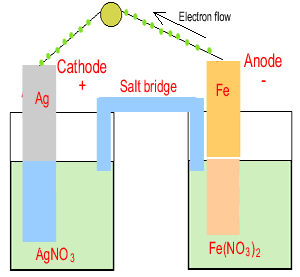Constructing an electrochemical cell from an overall equation We can construct an electrochemical cell from an overall equation. We must follow a few steps as outlined below. For example. Iron metal is placed in a solution of silver nitrate and reacts according to the equation below. Fe(s) + 2Ag+(aq) => 2Ag(s) + Fe2+(aq) Step 1 Obtain the two half equations. Reduction - Ag+(aq) + e => Ag(s) Oxidation - Fe(s) => Fe2+(aq) + 2e  Step 2 Construct the following half cells Ag+(aq) / Ag(s) and Fe(s) / Fe2+(aq) If a metal is present in the half cell use it as the electrode. Otherwise use inert carbon or platinum rods.1) Construct an eletrochemical cell from the overall equation given below. Cu(s) + 2Ag+(aq) => 2Ag(s) + Cu2+(aq) Solution 2) Construct an eletrochemical cell from the overall equation given below. 5Cu(s) + 2MnO4-(aq) + 16H+(aq) => 2Mn2+(aq) + 5Cu2+(aq) + 8H2O(l) Solution Continue with exercises of setting up electrochemical cells from half cells

Home